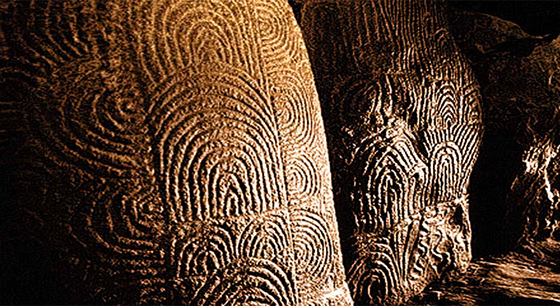# Happy Pi Day

The circumference of a circle, regardless of size is 3.14 times longer than the diameter (distance across). Circumference divided by diameter is a mathematical constant known as Pi. By definition Pi is a mathematical constant because it isn’t changed by the size of numbers it’s used to equate. Pi is irrational, meaning it has an infinite number of digits which never repeat. In 2018 physicist Peter Trueb calculated Pi to 22.4 trillion digits – 22,459,157,718,361 to be precise. Limitless as Pi may be, NASA’s Jet Propulsion Laboratory requires a mere 15 Pi digits to calculate interplanetary travel, mathematician James Grime argues 39 digits is enough to calculate circumference of the known universe. In 1988 physicist Larry Shaw organized the first Pi Day at San Francisco’s Exploratorium science museum on 3.14. In 2009 U.S. Congress officially recognized the fourteenth day of the third month as Pi Day.

Antiquity was no stranger to Pi, ancient Egypt and Babylon calculated approximations of Pi between 1900-1600 BC. In 250 BC Greek mathematician Archimedes is credited with the first algorithm calculating Pi as 3.14. Chinese mathematician Zu Chongzhi (429-501) calculated Pi to 6 decimal places.

March 14 ignites ponders of my Pi soft spot. Gavrinis, a Neolithic site inhabited between 5000-3500 BC on a tiny island off the Brittany coast of France. In the 1930’s French archeologists discovered a burial chamber on Gavrinis containing stone slabs with intricate carvings resembling fingerprints. Mathematicians saw it as indecipherable code, modern computer analysis blew our minds – Gavrinis code indicates the exact number of days in a year, reference to solstice and equinoxes, precise longitude/ latitude of the island, and the “mathematical constant Pi.Happy Pi Day.

https://notestoponder.wordpress.com/2013/09/29/gavrinis/http://www.geek.com